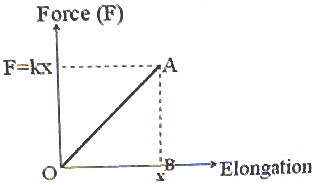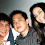## Pages

`“Life is like riding a bicycle.  To keep your balance you must keep moving.”–Albert Einstein`

## Saturday, November 15, 2008

### AP Physics B & C- Work, Energy & Power- Equations to be Remembered

You must remember the following points to make you strong in answering multiple choice questions involving work, energy and power:

(1) Work is done by a force if the point of application of the force is displaced. If the force vector F is constant and makes an angle θ with the displacement vector r, the work done (W) is given byW = Fr cos θ

In vector notation this is given by

W = F.r

Thus work is a scalar quantity given by the scalar product of force and displacement.

If the force is not constant and is a function of the displacement (as for instance, in compressing or elongating a spring), the work done is given by W = F.dr where dr is a small displacement for which the force F can be taken to be constant. The integration is to be carried out over the entire displacement. The work done by you in producing an elongation ‘x1 in a spring (which is not deformed initially) of force constant ‘k’ is given by

W = 0x1 F.dx = 0x1 kxdx = ½ kx12

[Note that the force with which you have to pull the spring to produce an extension ‘x’ is kx]

The work done in producing an extension ‘x’ in a spring is ½ kx2.

If the elongation of a spring is to be increased from x1 to x2 the work required is ½ k(x22 – x12).If the force and the displacement are in the same direction and the magnitude of the force varies with displacement, the work done is given by the area under the force-displacement graph obtained by plotting the displacement on the x-axis and the force on the y-axis (fig.).

In the case of a spring the force-elongation (displacement) curve is a straight line as shown in the figure. The work done in producing an elongation ‘x’ in a spring is the area under the curve (which is the area of the triangle OAB) and is equal to ½ Fx = ½ (kx)x = ½ kx2.(2) A force is conservative if the work done by the force in moving an object depends only on the initial and final positions of the object and is independent of the path followed between these positions. The total work done by a conservative force on an object is zero when it moves round any closed path and returns to the initial position. Gravtational force and electrostatic force are examples of conservative forces.

If the work done by a force on an object moving between two positions depends on the path taken, the force is called non-conservative force. Friction and visous force are non-conservative forces.

(3) Kinetic energy (K) of a body of mass m moving with velocity v is given by

K = ½ mv2

Since momentum, p = mv, we have

K = p2/2m

[Remember this useful expression].

(4) Work-energy theorem states that the work done by a force acting on a body is equal to the change in the kinetic energy of the body. If W is the work done and Ki and Kf are the initial and final kinetic energies respectively, we have

W = Kf Ki

(5) The gravitational potential energy of a body of mass m at a height h near the surface of the earth is mgh where g is the acceleration due to gravity at the place. Note that this energy is with respect to the reference level used for measuring the height and the value of h is negligible compared to the radius of the earth.

The change in the gravitational potential energy (Ug) of a mass m raised through a small height h can therefore be written as

Ug = mgh

You will find more details on gravitational potential energy in the posts dated 9th, 12th and 17th May 2008 which you can access by clicking on the label ‘gravitation’ below this post.

(6) When a spring is compressed or elongated, the work done on the spring is stored as elastic potential energy in the spring. The elastic potential energy in the spring which is elongated or compressed through a distance ‘x’ is ½ kx2.

(7) Law of conservation of energy states that energy can neither be created nor destroyed but can only be transferred from one form to another.

You should understand that the production of energy by annihilating mass in nuclear reactions does not violate the law of conservation of energy since mass itself is to be treated as a cocentrated form of energy in accordance with Einstein’s mass-energy relation, E = mc2.

(8) Power is the time rate at which work is done or energy is transferred.

The average power Pav = W/t where W is the total work done in a time t.

Instantaneous power P = dW/dt where dW is the work done in a very small time dt at the instant t.

(9) The instantaneous power can be expressed as the scalar product of the force vector F and the velocity vector v as

P = F.v

This is easily obtained since dW = F.dr so that P = dW/dt = F.(dr/dt) = F.v

(10) Elastic collision is one in which momentum and kinetic energy are conserved. Inelastic collision is one in which kinetic energy is not conserved, but momentum is conserved.

You should note that momentum is conserved in elastic as well as inelastic collisions; but kinetic energy is conserved in the case of elastic collisions only.

In a completely inelastic collision the two colliding bodies move together after the collision.If the mass m1 moves with velocity v1i in the positive x-direction and suffers a completely inelastic head on collision (collision in one dimension) with the mass m2 at rest (fig.), the common velocity (vf) with which the two masses will move is given by

m1v1i = (m1 + m2) vf, on applying the law of conservation of momentum.

Therefore, vf = m1v1i /(m1 + m2)

If the collision is elastic in the case of the above masses, we will have different

final velocities v1f and v2f for the two bodies after the collision, as given by the momentum conservationlaw:

m1v1i = m1 v1f + m2 v2f -------(i)

Since there are two unknowns (v1f and v2f) we require one more equation to solve for the unknowns and we have the kinetic energy equation,

½ m1v1i2 =½ m1 v1f 2+ ½ m2 v2f 2

Or, m1v1i2 = m1 v1f 2+ m2 v2f 2-------(ii)

Equations (i) and (ii) give

v1f = (m1 m2) v1i /(m1 + m2) and

v2f = 2 m1v1i /(m1 + m2)

If the the two bodies are of the same mass, the colliding mass m1 will come to rest and will hand over its velocity to the other mass m2 which was initially at rest. If m2>> m1, the velocity of m1 will get reversed and m2 will continue to remain at rest.

If m2 also has an initial velocity v2i you can incorporate its initial momentum and kinetic energy in the above equations and solve for the final velocities of the bodies. You will then get

v1f = [2 m2v2i + (m1 m2) v1i] /(m1 + m2) and

v2f = [2 m1v1i + (m2 m1) v2i] /(m1 + m2)

Try to derive these equations as an exercise.

In the next post we will discuss questions in this section. Meanwhile go through some useful multiple choice questions (with solution) here at physicsplus.

#### 1 comment:

1.a blog on physics! I haven't seen that before! I never liked physics like I should have when I was in college. I don't know why :(

Just dropping by! Keep up the good work! I bet there are many people especially physics students finding your site very useful!